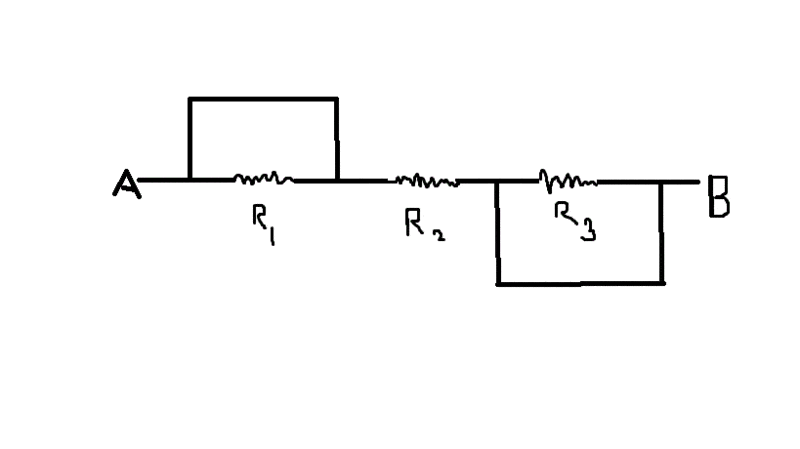# Current through a conductor of zero resistance

If a resistor shares common junctions to a conductor with no resistance in a circuit, will current flow through the resistor? Also, what is the equivalent resistance between A and B as in the figure...

#### Attachments

•Untitled.gif
6 KB · Views: 544

Nugatory
Mentor
If the wires were really perfect conductors with zero resistance, then there would be no voltage difference between the two ends of the resistor and therefore no current flow through the resistor.

In practice, no wire is a perfect conductor so there's always some tiny resistance and therefore some tiny voltage difference across the resistors to drive some tiny current flow through the resistor. However this current will be well and thoroughly negligible compared with the current through the wires (which will very quickly melt, burn, catch on fire, or explode if there's no fuse, internal resistance in the power supply, or some other current-limiting device).

Last edited:
if by the equivalent resistance you mean the total resistance it has with the junctions at both ends then the only resistance in your schematic is the one through "r2"

Nugatory
Mentor
What is the equivalent resistance between A and B in this case...

Try redrawing that picture so that the resistors are side by side on your sheet of paper... You'll find that this makes the problem much easier.

If the wires were really perfect conductors with zero resistance, then there would be no voltage difference between the two ends of the resistor and therefore no current flow through the resistor.

In practice, no wire is a perfect conductor so there's always some tiny resistance and therefore some tiny voltage difference across the resistors to drive some tiny current flow through the resistor. However this current will be well and thoroughly negligible compared with the current through the wires (which will very quickly melt, burn, catch on fire, or explode if there's no fuse, internal resistance in the power supply, or some other current-limiting device).

Where does superconductivity fit in this explanation? I understand that superconductors have zero resistance and currents do flow in suoerconductors

Try redrawing that picture so that the resistors are side by side on your sheet of paper... You'll find that this makes the problem much easier.

Can you explain it properly, perhaps upload the diagram you are referring to.......

Nugatory
Mentor
Where does superconductivity fit in this explanation? I understand that superconductors have zero resistance and currents do flow in suoerconductors

There is always some current-limiting device in such a setup, so infinite currents are not observed. But you are right that a superconductor can behave a lot more like an ideal wire than anything you're going to be able buy in a roll and solder together.

Last edited:
Nugatory
Mentor
Can you explain it properly, perhaps upload the diagram you are referring to.......

Not until we've given you a few more hints... This problem is much easier than it looks.... The end of every resistor in your diagram is connected directly to either point A or point B.

berkeman
Mentor
Can you explain it properly, perhaps upload the diagram you are referring to.......

Even though this is not directly homework, you still need to show some effort here....

Since the left end of R1 is shorted to the right end of R2, just rotate R1 up and over clockwise 180 degrees to put it in parallel with R2. Then do a similar thing with R3 -- rotate it clockwise 180 degrees to put in in parallel with R2. What does the diagram look like now?

There is always some current-limiting device in such a setup, so infinite currents are not observed. But you are right that a superconductor can behave a lot more like an ideal wire than anything you're going to be able buy in a roll and solder together.

It was not suggested that the current was infinite !!!
What 'current-limiting device'do you have in mind?

Last edited by a moderator:
berkeman
Mentor
Where does superconductivity fit in this explanation? I understand that superconductors have zero resistance and currents do flow in suoerconductors

It was not suggested that the current was infinite !!!
What 'current-limiting device'do you have in mind?

The maximum current that can be supported by a superconductor is limited either by the current density, or by the magnetic field generated by the current. Above those limits, the superconductor stops being a superconductor, develops some loss, and all hell breaks loose.

This paper from arxiv has some good info: http://www.google.com/url?sa=t&rct=...YReN1mhykYSVQvK0lVOkTmA&bvm=bv.46471029,d.cGE

.

SammyS
Staff Emeritus
Homework Helper
Gold Member
What is the equivalent resistance between A and B in this case...
As Crazymechanic already pointed out, the equivalent resistance between A and B is R2 .For the parallel portions of the circuit:

You can't use ##\displaystyle \ \frac{1}{R_\text{Parallel}}=\frac{1}{R_A}+\frac{1}{R_B} \,,\
## because one of the resistances is zero.

Instead, use ##\displaystyle \ R_\text{Parallel}=\frac{R_A\,R_B}{R_A+R_B} \ .##